# How to use Google Colaboratory for Video Processing

Did you know that a set of computer algorithms can process a video stream in a way that allows them to detect criminal activity, control traffic jams, and even automatically detect events in sports broadcasts? Thanks to the application of machine learning (ML), the idea of acquiring so much data from a simple video doesn’t seem that unrealistic. In this article, we will discuss about applying the pre-built logic of a machine learning algorithm for object detection and segmentation to a video.

In particular, we talk about how to configure Google Colaboratory for solving video processing tasks with machine learning. You’ll learn how to use this Google service and the free NVIDIA Tesla K80 GPU that it provides for achieving your own goals in training neural networks. This article will be useful for people who are getting familiar with machine learning and considering working with image recognition and video processing.

Image processing with limited hardware resources

The task is recognizing people in a video recording with the help of machine learning (ML) algorithms. We decided to begin with the basics. First, let’s consider what a video recording actually is.

From a technical point of view, any video recording consists of a series of still images in a particular format that is compressed with a video codec. Consequently, object recognition on a video stream comes down to splitting the stream into separate images, or frames, and applying a pre-trained ML image recognition algorithm to them.

To do this, we decided to use a neural network from the Mask_R-CNN repository for classifying single images. The repository contains an implementation of a convolutional neural network on Python3, TensorFlow, and Keras. Let’s see what came out of this plan.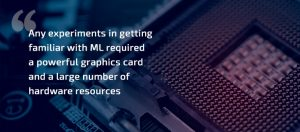We developed and implemented a simple sample of Mask_RCNN that received a picture as the input and recognized objects in it. We created a sample on the basis of the demo.ipynb description is taken from the Mask_R-CNN repository. Here’s the code of our sample:

 `import` `os, sys ` `import` `random ` `import` `math ` `import` `numpy as np ` `import` `skimage.io ` `import` `matplotlib ` `import` `matplotlib.pyplot as plt ` `   `  `os.chdir(``"/content/drive/My Drive/Colab Notebooks/MRCNN_pure"``) ` `sys.path.append(``"/content/drive/My Drive/Colab Notebooks/MRCNN_pure"``) ` `   `  `# Root directory of the project ` `ROOT_DIR ``=` `os.path.abspath(``"."``) ` `   `  `# Import Mask RCNN ` `sys.path.append(ROOT_DIR)  ``# To find local version of the library ` ` `  `from` `mrcnn ``import` `utils ` `import` `mrcnn.model as modellib ` `from` `mrcnn ``import` `visualize ` ` `  `# Import COCO config ` `sys.path.append(os.path.join(ROOT_DIR, ``"samples/coco/"``))  ``# To find local version ` `import` `coco ` `   `  `# Directory to save logs and trained model ` `MODEL_DIR ``=` `os.path.join(ROOT_DIR, ``"logs"``) ` `   `  `# Local path to trained weights file ` `COCO_MODEL_PATH ``=` `os.path.join(ROOT_DIR, ``"mask_rcnn_coco.h5"``) ` `# Download COCO trained weights from Releases if needed ` `if` `not` `os.path.exists(COCO_MODEL_PATH): ` `    ``utils.download_trained_weights(COCO_MODEL_PATH) ` `   `  `# Directory of images to run detection on ` `IMAGE_DIR ``=` `os.path.join(ROOT_DIR, ``"images"``) ` `   `  `class` `InferenceConfig(coco.CocoConfig): ` `    ``# Set batch size to 1 since we'll be running inference on ` `    ``# one image at a time. Batch size = GPU_COUNT * IMAGES_PER_GPU ` `    ``GPU_COUNT ``=` `1` `    ``IMAGES_PER_GPU ``=` `1` `   `  `config ``=` `InferenceConfig() ` `config.display() ` `   `  `# Create model object in inference mode. ` `model ``=` `modellib.MaskRCNN(mode``=``"inference"``, model_dir``=``MODEL_DIR, config``=``config) ` `   `  `# Load weights trained on MS-COCO ` `model.load_weights(COCO_MODEL_PATH, by_name``=``True``) ` `   `  `# COCO Class names ` `# Index of the class in the list is its ID. For example, to get ID of ` `# the teddy bear class, use: class_names.index('teddy bear') ` `class_names ``=` `[``'BG'``, ``'person'``, ``'bicycle'``, ``'car'``, ``'motorcycle'``, ``'airplane'``, ` `               ``'bus'``, ``'train'``, ``'truck'``, ``'boat'``, ``'traffic light'``, ` `               ``'fire hydrant'``, ``'stop sign'``, ``'parking meter'``, ``'bench'``, ``'bird'``, ` `               ``'cat'``, ``'dog'``, ``'horse'``, ``'sheep'``, ``'cow'``, ``'elephant'``, ``'bear'``, ` `               ``'zebra'``, ``'giraffe'``, ``'backpack'``, ``'umbrella'``, ``'handbag'``, ``'tie'``, ` `               ``'suitcase'``, ``'frisbee'``, ``'skis'``, ``'snowboard'``, ``'sports ball'``, ` `               ``'kite'``, ``'baseball bat'``, ``'baseball glove'``, ``'skateboard'``, ` `               ``'surfboard'``, ``'tennis racket'``, ``'bottle'``, ``'wine glass'``, ``'cup'``, ` `               ``'fork'``, ``'knife'``, ``'spoon'``, ``'bowl'``, ``'banana'``, ``'apple'``, ` `               ``'sandwich'``, ``'orange'``, ``'broccoli'``, ``'carrot'``, ``'hot dog'``, ``'pizza'``, ` `               ``'donut'``, ``'cake'``, ``'chair'``, ``'couch'``, ``'potted plant'``, ``'bed'``, ` `               ``'dining table'``, ``'toilet'``, ``'tv'``, ``'laptop'``, ``'mouse'``, ``'remote'``, ` `               ``'keyboard'``, ``'cell phone'``, ``'microwave'``, ``'oven'``, ``'toaster'``, ` `               ``'sink'``, ``'refrigerator'``, ``'book'``, ``'clock'``, ``'vase'``, ``'scissors'``, ` `               ``'teddy bear'``, ``'hair drier'``, ``'toothbrush'``] ` `   `  `# Load a random image from the images folder ` `file_names ``=` `next``(os.walk(IMAGE_DIR))[``2``] ` `image ``=` `skimage.io.imread(os.path.join(IMAGE_DIR, random.choice(file_names))) ` `   `  `# Run detection ` `results ``=` `model.detect([image], verbose``=``1``) ` `   `  `# Visualize results ` `r ``=` `results[``0``] ` `visualize.display_instances(image, r[``'rois'``], r[``'masks'``],  ` `                            ``r[``'class_ids'``],class_names, r[``'scores'``]) `

In this sample, /content/drive/My Drive/Colab Notebooks/MRCNN_pure is the path to our repository with Mask_R-CNN. As a result, we got the following: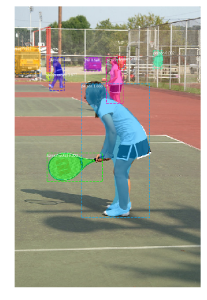This part of the demo code looks through the images folder, randomly selects an image, and loads it to our neural network model for classification:

 `# Load a random image from the images folder ` `file_names ``=` `next``(os.walk(IMAGE_DIR))[``2``] ` `image ``=` `skimage.io.imread(os.path.join(IMAGE_DIR, random.choice(file_names))) ` ` `  `# Run detection ` `results ``=` `model.detect([image], verbose ``=` `1``) `

Let’s modify the Mask_R-CNN sample to make it recognize all images in the images folder:

 `# Load a random image from the images folder ` `file_names ``=` `next``(os.walk(IMAGE_DIR))[``2``] ` `   `  `for` `file_name ``in` `file_names: ` `    ``image ``=` `skimage.io.imread(os.path.join(IMAGE_DIR, file_name)) ` `   `  `    ``# Run detection ` `    ``results ``=` `model.detect([image], verbose ``=` `1``) ` `   `  `    ``# Visualize results ` `    ``r ``=` `results[``0``] ` `    ``visualize.display_instances(image, r[``'rois'``], r[``'masks'``], ` `                         ``r[``'class_ids'``],class_names, r[``'scores'``]) `

After running the demo code for five minutes, the console displayed the following output:

Processing 1 images
image shape: (415, 640, 3) min: 0.00000 max: 255.00000 uint8
molded_images shape: (1, 1024, 1024, 3) min: -123.70000 max: 151.10000 float64
image_metas shape: (1, 93) min: 0.00000 max: 1024.00000 float64
anchors shape: (1, 261888, 4) min: -0.35390 max: 1.29134 float32
Segmentation fault (core dumped)

Initially, we ran the demo code on a computer with an Intel Core i5 and 8GB of RAM without a discrete graphics card. The code crashed each time in different places, but most often it crashed in the TensorFlow framework during memory allocation. Moreover, any attempts to run any other software during the image recognition process slowed down the computer to the point of being useless.

Thus, we faced a serious problem: any experiments in getting familiar with ML required a powerful graphics card and more hardware resources. Without this, we couldn’t run any other tasks while recognizing a large number of images.

Increasing our hardware resources with Google Colaboratory

We decided to expand our hardware resources by using the Colaboratory service by Google, which is also known as Colab. Google Colab is a free cloud service that provides use of a CPU and GPU as well as a preconfigured virtual machine instance. Specifically, Google offers the NVIDIA Tesla K80 GPU with 12GB of dedicated video memory, which makes Colab a perfect tool for experimenting with neural networks.

Before explaining how to work with this Google service, we’d like to underline other beneficial Colaboratory features.

By choosing Colab for your ML experiments, you’ll get:

– support for Python 2.7 and Python 3.6 so you can improve your coding skills;
– the ability to work with Jupyter notebook so you can create, edit, and share your .ipynb files;
– the ability to connect to a Jupyter runtime using your local machine;
– many pre-installed libraries including TensorFlow, Keras, and OpenCV, as well as the possibility to interact with your custom libraries in Google Colaboratory;
– integration with GitHub so you can load public GitHub notebooks or save a copy of your Colab file to GitHub;
– simple visualization with such popular libraries as matplotlib;
forms that can be used to parameterize code;

In order to start using the Google Colab GPU, you just need to provide access to your .ipynb script that’s implemented in a Docker container. The Docker container is assigned to you only for 12 hours. All scripts created by you are stored by default in your Google Drive in the Colab Notebooks section, which is automatically created as you connect to Colaboratory. After the expiry of 12 hours, all of your data in the container will be deleted. You can avoid this by mounting your Google Drive in your container and working with it. Otherwise, the file system of the Docker image will be available only for a limited period of time.

Let’s first explain how you can create your .ipynb notebook. Open Google Colaboratory here, select the Google Drive section, and click NEW PYTHON 3 NOTEBOOK: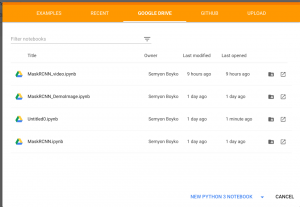Rename your notebook whatever you want by clicking on the file name. Now you need to choose your hardware. To do this, just go to the Edit section, find Notebook Settings, select GPU as the Hardware accelerator, and save changes by clicking SAVE.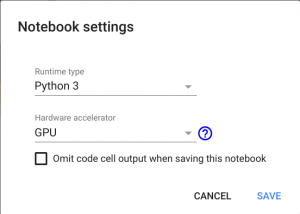After the new settings are saved, a Docker container with a discrete graphics card will become available to you. You’ll be notified about this with a “Connected” message in the upper right of the page: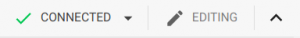If you don’t see this message, select Connect to hosted runtime.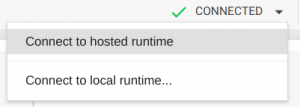Now you can mount your Google Drive to this container in order to relocate the source code and save the results of your work in the container. To do this, just copy the code below in the first table cell and press the Play button (or Shift + Enter).

Mount Google Drive by running this code:

 `# from google.colab import drive ` `drive.mount(``'/content/drive'``) `

You’ll get a request for authorization. Click the link, authorize, copy the verification code, paste it in the text box in your .ipynb script, and press Enter. If authorization is successful, your Google Drive will be mounted under the path /content/drive/My Drive. To follow the file tree, select Files in the left-hand menu.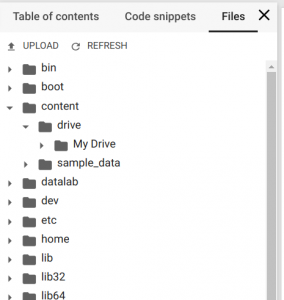Now you have a Docker container with the Tesla K80 GPU, your Google Drive as file storage, and the .ipynb notebook for script execution.

Using Google Colab for object recognition

Now we’ll describe how to run our Mask_R-CNN sample for object recognition in Google Colab. We upload the Mask_RCNN repository to our Google Drive following the /content/drive/My Drive/Colab Notebooks/ path.

Then we add our sample code to the .ipynb script. When you do this, don’t forget to change your path to the Mask_RCNN folder like this:

 `os.chdir(``"/content/drive/My Drive/Colab Notebooks/MRCNN_pure"``) ` `sys.path.append(``"/content/drive/My Drive/Colab Notebooks/MRCNN_pure"``) `

If you do everything right, the results of code execution will provide you with an image where all objects are detected and recognized.

You can also modify the sample code to make it process all of the test images:

 `import os, sys ` `import random ` `import math ` `import numpy as np ` `import skimage.io ` `import matplotlib ` `import matplotlib.pyplot as plt ` `   `  `os.chdir(``"/content/drive/My Drive/Colab Notebooks/MRCNN_pure"``) ` `sys.path.append(``"/content/drive/My Drive/Colab Notebooks/MRCNN_pure"``) ` `   `  `# Root directory of the project ` `ROOT_DIR = os.path.abspath(``"."``) ` `   `  `# Import Mask RCNN ` `sys.path.append(ROOT_DIR)  # To find local version of the library ` `from mrcnn import utils ` `import mrcnn.model as modellib ` `from mrcnn import visualize ` `# Import COCO config ` `sys.path.append(os.path.join(ROOT_DIR, ``"samples/coco/"``))  # To find local version ` `import coco ` `   `  `# Directory to save logs and trained model ` `MODEL_DIR = os.path.join(ROOT_DIR, ``"logs"``) ` `   `  `# Local path to trained weights file ` `COCO_MODEL_PATH = os.path.join(ROOT_DIR, ``"mask_rcnn_coco.h5"``) ` `# Download COCO trained weights from Releases if needed ` `if` `not os.path.exists(COCO_MODEL_PATH): ` `    ``utils.download_trained_weights(COCO_MODEL_PATH) ` `   `  `# Directory of images to run detection on ` `IMAGE_DIR = os.path.join(ROOT_DIR, ``"images"``) ` `   `  `class` `InferenceConfig(coco.CocoConfig): ` `    ``# Set batch size to 1 since we'll be running inference on ` `    ``# one image at a time. Batch size = GPU_COUNT * IMAGES_PER_GPU ` `    ``GPU_COUNT = 1 ` `    ``IMAGES_PER_GPU = 1 ` `   `  `config = InferenceConfig() ` `config.display() ` `   `  `# Create model object in inference mode. ` `model = modellib.MaskRCNN(mode=``"inference"``, model_dir=MODEL_DIR, config=config) ` `   `  `# Load weights trained on MS-COCO ` `model.load_weights(COCO_MODEL_PATH, by_name=True) ` `   `  `# COCO Class names ` `# Index of the class in the list is its ID. For example, to get ID of ` `# the teddy bear class, use: class_names.index('teddy bear') ` `class_names = [``'BG'``, ``'person'``, ``'bicycle'``, ``'car'``, ``'motorcycle'``, ``'airplane'``, ` `               ``'bus'``, ``'train'``, ``'truck'``, ``'boat'``, ``'traffic light'``, ` `               ``'fire hydrant'``, ``'stop sign'``, ``'parking meter'``, ``'bench'``, ``'bird'``, ` `               ``'cat'``, ``'dog'``, ``'horse'``, ``'sheep'``, ``'cow'``, ``'elephant'``, ``'bear'``, ` `               ``'zebra'``, ``'giraffe'``, ``'backpack'``, ``'umbrella'``, ``'handbag'``, ``'tie'``, ` `               ``'suitcase'``, ``'frisbee'``, ``'skis'``, ``'snowboard'``, ``'sports ball'``, ` `               ``'kite'``, ``'baseball bat'``, ``'baseball glove'``, ``'skateboard'``, ` `               ``'surfboard'``, ``'tennis racket'``, ``'bottle'``, ``'wine glass'``, ``'cup'``, ` `               ``'fork'``, ``'knife'``, ``'spoon'``, ``'bowl'``, ``'banana'``, ``'apple'``, ` `               ``'sandwich'``, ``'orange'``, ``'broccoli'``, ``'carrot'``, ``'hot dog'``, ``'pizza'``, ` `               ``'donut'``, ``'cake'``, ``'chair'``, ``'couch'``, ``'potted plant'``, ``'bed'``, ` `               ``'dining table'``, ``'toilet'``, ``'tv'``, ``'laptop'``, ``'mouse'``, ``'remote'``, ` `               ``'keyboard'``, ``'cell phone'``, ``'microwave'``, ``'oven'``, ``'toaster'``, ` `               ``'sink'``, ``'refrigerator'``, ``'book'``, ``'clock'``, ``'vase'``, ``'scissors'``, ` `               ``'teddy bear'``, ``'hair drier'``, ``'toothbrush'``] ` `   `  `# Load a random image from the images folder ` `file_names = next(os.walk(IMAGE_DIR)) ` `   `  `for` `file_name in file_names: ` `    ``image = skimage.io.imread(os.path.join(IMAGE_DIR, file_name)) ` `   `  `    ``# Run detection ` `    ``results = model.detect([image], verbose=1) ` `   `  `    ``# Visualize results ` `    ``r = results ` `    ``visualize.display_instances(image, r[``'rois'``], r[``'masks'``],  ` `                      ``r[``'class_ids'``],class_names, r[``'scores'``]) `

Using object detection in Google Colab, we received the results with recognized objects quickly, while our computer continued to perform as usual even during the image recognition process.

Using Google Colab for video processing

Let’s see how we applied this method for recognizing people in a video stream. We uploaded a test video file to our Google Drive. To train our script to work with a video stream, we used OpenCV, a popular open source computer vision library.

We don’t need the whole code that read and implemented the recognition model on one image. So instead of opening the video file, we run the video stream and move its pointer to the 1, 000th frame as there are no objects to recognize in the intro of the recording.

 `import cv2 ` `... ` `VIDEO_STREAM = ``"/content/drive/My Drive/Colab Notebooks/Millery.avi"` `VIDEO_STREAM_OUT = ``"/content/drive/My Drive/Colab Notebooks/Result.avi"` `... ` ` `  `# initialize the video stream and pointer to output video file ` `vs = cv2.VideoCapture(VIDEO_STREAM) ` `writer = None ` `vs.set(cv2.CAP_PROP_POS_FRAMES, 1000); `

Then we process 20, 000 frames with our neural network model. The OpenCV object allows us to get images by frame from the video file using the read() method. The received image is passed to the model.detect() method and the results are visualized with the visualize.display_instances() function.

However, we faced a problem: the display_instances() function from the Mask_RCNN repository reflects detected objects in the image, but the image doesn’t returned. We decided to simplify the display_instances() function and make it return the image with displayed objects:

 `def` `display_instances(image, boxes, masks, ids, names, scores): ` `    ``""" ` `        ``take the image and results and apply the mask, box, and Label ` `    ``"""` `    ``n_instances ``=` `boxes.shape[``0``] ` `    ``colors ``=` `visualize.random_colors(n_instances) ` `   `  `    ``if` `not` `n_instances: ` `        ``print``(``'NO INSTANCES TO DISPLAY'``) ` `    ``else``: ` `        ``assert` `boxes.shape[``0``] ``=``=` `masks.shape[``-``1``] ``=``=` `ids.shape[``0``] ` `   `  `    ``for` `i, color ``in` `enumerate``(colors): ` `        ``if` `not` `np.``any``(boxes[i]): ` `            ``continue` `   `  `        ``y1, x1, y2, x2 ``=` `boxes[i] ` `        ``label ``=` `names[ids[i]] ` `        ``score ``=` `scores[i] ``if` `scores ``is` `not` `None` `else` `None` `        ``caption ``=` `'{} {:.2f}'``.``format``(label, score) ``if` `score ``else` `label ` `        ``mask ``=` `masks[:, :, i] ` `   `  `        ``image ``=` `visualize.apply_mask(image, mask, color) ` `        ``image ``=` `cv2.rectangle(image, (x1, y1), (x2, y2), color, ``2``) ` `        ``image ``=` `cv2.putText( ` `            ``image, caption, (x1, y1), cv2.FONT_HERSHEY_COMPLEX, ``0.7``, color, ``2` `        ``) ` `   `  `    ``return` `image `

After processing, the frames should be bound back together into a new video file. We can also do this with the OpenCV library. All we need to do is allocate the VideoWriter object from the OpenCV library:

 `fourcc ``=` `cv2.VideoWriter_fourcc(``*``"XVID"``) ` `writer ``=` `cv2.VideoWriter(VIDEO_STREAM_OUT, fourcc, ``30``,  ` `             ``(masked_frame.shape[``1``], masked_frame.shape[``0``]), ``True``) `

using the type of video we provided for the input. We get the video file type with the help of the ffprobe command:

ffprobe Result.avi

Duration: N/A, start: 0.000000, bitrate: N/A
Stream #0:0: Video: mpeg4 (Simple Profile) (XVID / 0x44495658), yuv420p, 640×272 [SAR 1:1 DAR 40:17], 30 fps, 30 tbr, 30 tbn, 30 tbc

At the beginning of the script, we need to specify paths to the target video files for processing: VIDEO_STREAM and VIDEO_STREAM_OUT.

Here’s the full script that we developed for video recognition:

 `from` `google.colab ``import` `drive ` `drive.mount(``'/content/drive'``) ` `   `  `import` `os, sys ` `import` `random ` `import` `math ` `import` `numpy as np ` `import` `skimage.io ` `import` `matplotlib ` `import` `matplotlib.pyplot as plt ` `import` `cv2 ` `   `  `from` `matplotlib.patches ``import` `Polygon ` `   `  `os.chdir(``"/content/drive/My Drive/Colab Notebooks/MRCNN_pure"``) ` `sys.path.append(``"/content/drive/My Drive/Colab Notebooks/MRCNN_pure"``) ` `VIDEO_STREAM ``=` `"/content/drive/My Drive/Colab Notebooks/Millery.avi"` `VIDEO_STREAM_OUT ``=` `"/content/drive/My Drive/Colab Notebooks/Result.avi"` `   `  `# Root directory of the project ` `ROOT_DIR ``=` `os.path.abspath(``"."``) ` `   `  `# Import Mask RCNN ` `sys.path.append(ROOT_DIR)  ``# To find local version of the library ` `from` `mrcnn ``import` `utils ` `import` `mrcnn.model as modellib ` `from` `mrcnn ``import` `visualize ` `# Import COCO config ` `sys.path.append(os.path.join(ROOT_DIR, ``"samples/coco/"``))  ``# To find local version ` `import` `coco ` `   `  `# Directory to save logs and trained model ` `MODEL_DIR ``=` `os.path.join(ROOT_DIR, ``"logs"``) ` `   `  `# Local path to trained weights file ` `COCO_MODEL_PATH ``=` `os.path.join(ROOT_DIR, ``"mask_rcnn_coco.h5"``) ` `# Download COCO trained weights from Releases if needed ` `if` `not` `os.path.exists(COCO_MODEL_PATH): ` `    ``utils.download_trained_weights(COCO_MODEL_PATH) ` `   `  `# Directory of images to run detection on ` `IMAGE_DIR ``=` `os.path.join(ROOT_DIR, ``"images"``) ` `   `  `class` `InferenceConfig(coco.CocoConfig): ` `    ``# Set batch size to 1 since we'll be running inference on ` `    ``# one image at a time. Batch size = GPU_COUNT * IMAGES_PER_GPU ` `    ``GPU_COUNT ``=` `1` `    ``IMAGES_PER_GPU ``=` `1` `       `  `       `  `def` `display_instances(image, boxes, masks, ids, names, scores): ` `    ``""" ` `        ``take the image and results and apply the mask, box, and Label ` `    ``"""` `    ``n_instances ``=` `boxes.shape[``0``] ` `    ``colors ``=` `visualize.random_colors(n_instances) ` `   `  `    ``if` `not` `n_instances: ` `        ``print``(``'NO INSTANCES TO DISPLAY'``) ` `    ``else``: ` `        ``assert` `boxes.shape[``0``] ``=``=` `masks.shape[``-``1``] ``=``=` `ids.shape[``0``] ` `   `  `    ``for` `i, color ``in` `enumerate``(colors): ` `        ``if` `not` `np.``any``(boxes[i]): ` `            ``continue` `   `  `        ``y1, x1, y2, x2 ``=` `boxes[i] ` `        ``label ``=` `names[ids[i]] ` `        ``score ``=` `scores[i] ``if` `scores ``is` `not` `None` `else` `None` `        ``caption ``=` `'{} {:.2f}'``.``format``(label, score) ``if` `score ``else` `label ` `        ``mask ``=` `masks[:, :, i] ` `   `  `        ``image ``=` `visualize.apply_mask(image, mask, color) ` `        ``image ``=` `cv2.rectangle(image, (x1, y1), (x2, y2), color, ``2``) ` `        ``image ``=` `cv2.putText( ` `            ``image, caption, (x1, y1), cv2.FONT_HERSHEY_COMPLEX, ``0.7``, color, ``2` `        ``) ` `   `  `    ``return` `image ` `   `  `   `  `config ``=` `InferenceConfig() ` `config.display() ` `   `  `# Create model object in inference mode. ` `model ``=` `modellib.MaskRCNN(mode``=``"inference"``, model_dir``=``MODEL_DIR, config``=``config) ` `   `  `# Load weights trained on MS-COCO ` `model.load_weights(COCO_MODEL_PATH, by_name``=``True``) ` `   `  `# COCO Class names ` `# Index of the class in the list is its ID. For example, to get ID of ` `# the teddy bear class, use: class_names.index('teddy bear') ` `class_names ``=` `[``'BG'``, ``'person'``, ``'bicycle'``, ``'car'``, ``'motorcycle'``, ``'airplane'``, ` `               ``'bus'``, ``'train'``, ``'truck'``, ``'boat'``, ``'traffic light'``, ` `               ``'fire hydrant'``, ``'stop sign'``, ``'parking meter'``, ``'bench'``, ``'bird'``, ` `               ``'cat'``, ``'dog'``, ``'horse'``, ``'sheep'``, ``'cow'``, ``'elephant'``, ``'bear'``, ` `               ``'zebra'``, ``'giraffe'``, ``'backpack'``, ``'umbrella'``, ``'handbag'``, ``'tie'``, ` `               ``'suitcase'``, ``'frisbee'``, ``'skis'``, ``'snowboard'``, ``'sports ball'``, ` `               ``'kite'``, ``'baseball bat'``, ``'baseball glove'``, ``'skateboard'``, ` `               ``'surfboard'``, ``'tennis racket'``, ``'bottle'``, ``'wine glass'``, ``'cup'``, ` `               ``'fork'``, ``'knife'``, ``'spoon'``, ``'bowl'``, ``'banana'``, ``'apple'``, ` `               ``'sandwich'``, ``'orange'``, ``'broccoli'``, ``'carrot'``, ``'hot dog'``, ``'pizza'``, ` `               ``'donut'``, ``'cake'``, ``'chair'``, ``'couch'``, ``'potted plant'``, ``'bed'``, ` `               ``'dining table'``, ``'toilet'``, ``'tv'``, ``'laptop'``, ``'mouse'``, ``'remote'``, ` `               ``'keyboard'``, ``'cell phone'``, ``'microwave'``, ``'oven'``, ``'toaster'``, ` `               ``'sink'``, ``'refrigerator'``, ``'book'``, ``'clock'``, ``'vase'``, ``'scissors'``, ` `               ``'teddy bear'``, ``'hair drier'``, ``'toothbrush'``] ` `   `  `# Initialize the video stream and pointer to output video file ` `vs ``=` `cv2.VideoCapture(VIDEO_STREAM) ` `writer ``=` `None` `vs.``set``(cv2.CAP_PROP_POS_FRAMES, ``1000``); ` `   `  `i ``=` `0` `while` `i < ``20000``: ` `  ``# read the next frame from the file ` `  ``(grabbed, frame) ``=` `vs.read() ` `  ``i ``+``=` `1` `    `  `  ``# If the frame was not grabbed, then we have reached the end ` `  ``# of the stream ` `  ``if` `not` `grabbed: ` `    ``print` `(``"Not grabbed."``) ` `    ``break``; ` `     `  `  ``# Run detection ` `  ``results ``=` `model.detect([frame], verbose``=``1``) ` `   `  `  ``# Visualize results ` `  ``r ``=` `results[``0``] ` `  ``masked_frame ``=` `display_instances(frame, r[``'rois'``], r[``'masks'``], r[``'class_ids'``], ` `                            ``class_names, r[``'scores'``]) ` `     `  `  ``# Check if the video writer is None ` `  ``if` `writer ``is` `None``: ` `    ``# Initialize our video writer ` `    ``fourcc ``=` `cv2.VideoWriter_fourcc(``*``"XVID"``) ` `    ``writer ``=` `cv2.VideoWriter(VIDEO_STREAM_OUT, fourcc, ``30``, ` `      ``(masked_frame.shape[``1``], masked_frame.shape[``0``]), ``True``) ` `    `  `  ``# Write the output frame to disk ` `  ``writer.write(masked_frame) ` `     `  `# Release the file pointers ` `print``(``"[INFO] cleaning up..."``) ` `writer.release() `

Conclusion:

Use a free Tesla K80 GPU provided by Google Colab